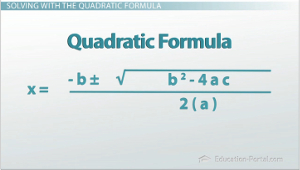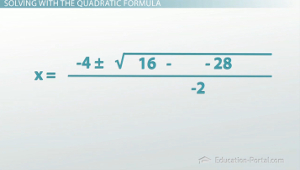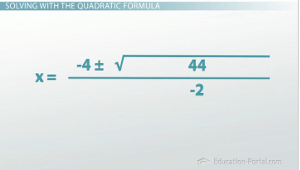# How to Use the Quadratic Formula to Solve a Quadratic Equation

An error occurred trying to load this video.

Try refreshing the page, or contact customer support.

Coming up next: How to Solve Quadratics That Are Not in Standard Form

### You're on a roll. Keep up the good work!

Replay
Your next lesson will play in 10 seconds
• 1:40 The Quadratic Formula
• 1:55 Standard Form
• 2:17 Identifying a, b and c
• 2:37 Using the Quadratic Formula
• 7:13 Using a Negative b Value
• 8:53 Lesson Summary

Want to watch this again later?

Timeline
Autoplay
Autoplay
Speed

#### Recommended Lessons and Courses for You

Lesson Transcript
Instructor: Luke Winspur

Luke has taught high school algebra and geometry, college calculus, and has a master's degree in education.

When solving a quadratic equation by factoring doesn't work, the quadratic formula is here to save the day. Learn what it is and how to use it in this lesson.

## Solving with the Quadratic FormulaYou just passed level one of Furious Fowls, the game that asks you to launch birds across the screen to get back at those pesky pigs that stole your eggs. You learned that we could figure out where our bird would hit the ground by solving the given quadratic equation where it equals 0. That required us to factor the equation and then use the zero product property to determine which two values would give us 0.

But now we're on to level two! It's going to get harder, but your goal will still be the same: use the equation given to you on the screen to make sure that your bird will hit its target on the first try. Alright, so here we go; let's go ahead and make a guess at where we'll need to launch our bird this time. I think this looks about right. Now, let's check our equation to see if this will actually work out or not.

The game tells us that our bird will fly in a parabola given by the equation y = -x2 + 4x + 7. Since we're again shooting for a pig on the ground, we're curious where this equation equals 0, so let's go ahead and substitute that in. Okay, now we need to try to factor in order to find x. Let's divide out the negative, leaving us with this: 0 = -(x2 - 4x - 7). Now look for a pair of numbers that have a product of -7 and a sum of -4. Hmmm, this isn't looking good. I don't think this trinomial can be factored. So, what now? In level one this worked every time, so we never had to do anything else! Looks like we're going to have to learn some new skills.

### The Quadratic Formula

Situations just like this, where we're trying to solve a quadratic that is unfactorable, is what the quadratic formula was made for! It's a little long and messy, but if you can remember it, you'll be able to solve any quadratic equation out there!### The Standard Form

You'll have to first remember the standard form of a quadratic equation, y = ax2 + bx + c. This will tell you what your a, b and c values are, but then it simply becomes a matter of putting the numbers into the right spots. Let's see if we can successfully do this to pass the second level of Furious Fowls.

### Identifying A, B and C

Going back to the original equation and identifying a, b and c should be our first step. A is in front of the x2, where there's only a negative symbol. That means that a is just -1. B is the number in front of the xs, which makes it 4, and c is the constant on the end, 7.

### Using the Quadratic Formula

Now, we can substitute these values into the quadratic formula and evaluate the resulting expression to find our answers! While this is theoretically pretty straightforward, there are quite a few spots that can easily trip you up. Because this is our first time, let's evaluate this one step by step together and go over some of the common mistakes. We're going to have to remember our order of operations and make sure we also keep track of all our negative signs.

For this problem, then, the first thing we'll have to take care of is the exponent we see. The exponent I see is a 2, which means a square, which means multiply that number by itself, so we do 4 * 4 to get 16. Next in the order of operations will come multiplication. Doing the multiplication on the inside of the square root (4 * -1 * 7) gives us -28, then doing the multiplication on the bottom of the fraction (2 * -1) is simply -2. We're now left with x = (-4 +/- √(16 - (-28))) / -2, and we're going to focus on the inside of the square root in the top of the fraction.

The inside of the square root in the formula is b2 - 4ac. This part is one of the hardest parts to evaluate and one of the easiest parts to make a mistake on. It also has a special name; it's called the discriminant. It has some important features that we'll talk about in other lessons, but for this lesson, you just need to know to be careful when evaluating it, specifically for the reason I'm about to mention. Notice that we ended up with a -28 after we did the multiplication, but I still have 16 - -28. Minus a negative is plus a positive, so 16 + 28 gives us 44.Now that we have the square root of 44, we'd like to check to see if we can take the square root of 44 nicely. Is there a number that, multiplied by itself, gives us 44? Well, 7 * 7 is 49, which is pretty close, but not quite, and 6*6 is 36, so again, not quite. So, the square root of 44 is an irrational number; it's going to be a really long decimal. That means that what we have is actually a pretty good answer, but there are two mistakes that students tend to make without realizing that this is an okay answer. One of those mistakes is to divide the -4 by the -2 to get 2 +/- the square root of 44. This is not allowed because the + root 44 kind of gets in the way of you doing that division.

To unlock this lesson you must be a Study.com Member.

### Register to view this lesson

Are you a student or a teacher?

### Unlock Your Education

#### See for yourself why 30 million people use Study.com

##### Become a Study.com member and start learning now.
Back
What teachers are saying about Study.com

### Earning College Credit

Did you know… We have over 160 college courses that prepare you to earn credit by exam that is accepted by over 1,500 colleges and universities. You can test out of the first two years of college and save thousands off your degree. Anyone can earn credit-by-exam regardless of age or education level.